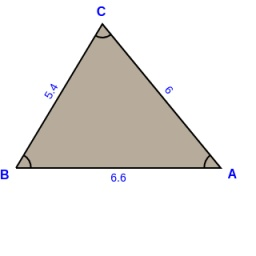# Following 6660

KLM triangle on sides k = 5.4 cm. L = 6 cm, m = 6.6 cm.
Construct a triangle K 'L'M 'for which the following holds: ∆KLM ~ ∆K 'L'M' and m '= 9.9cm

k1 =  8.1 cm
l1 =  9 cm
m1 =  9.9 cm

### Step-by-step explanation:

Try calculation via our triangle calculator.Did you find an error or inaccuracy? Feel free to write us. Thank you!

Tips for related online calculators